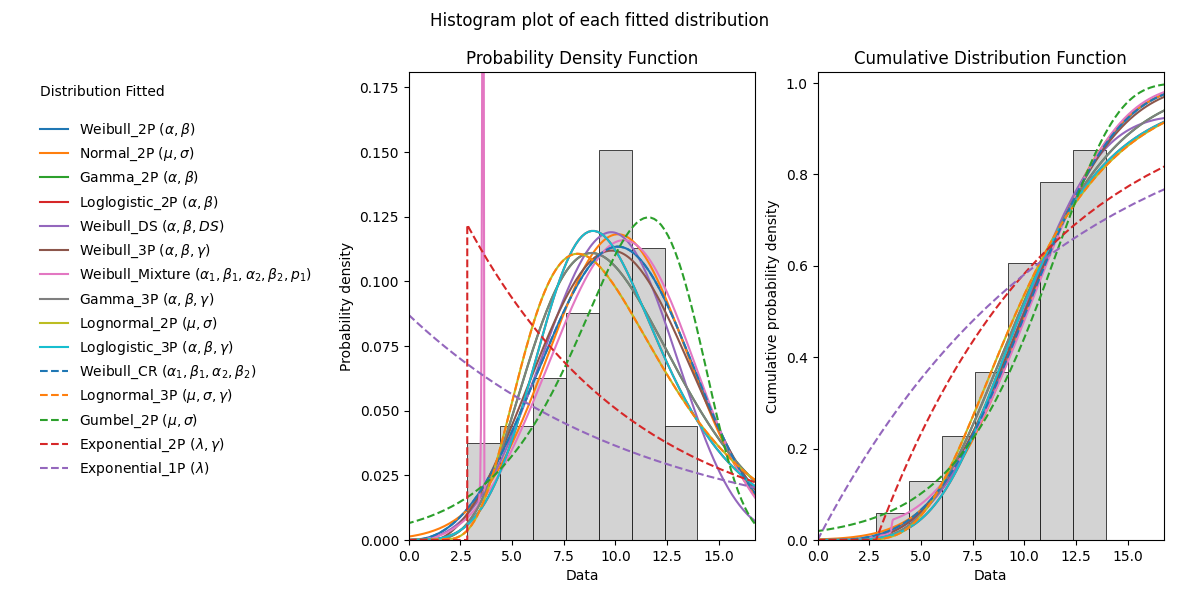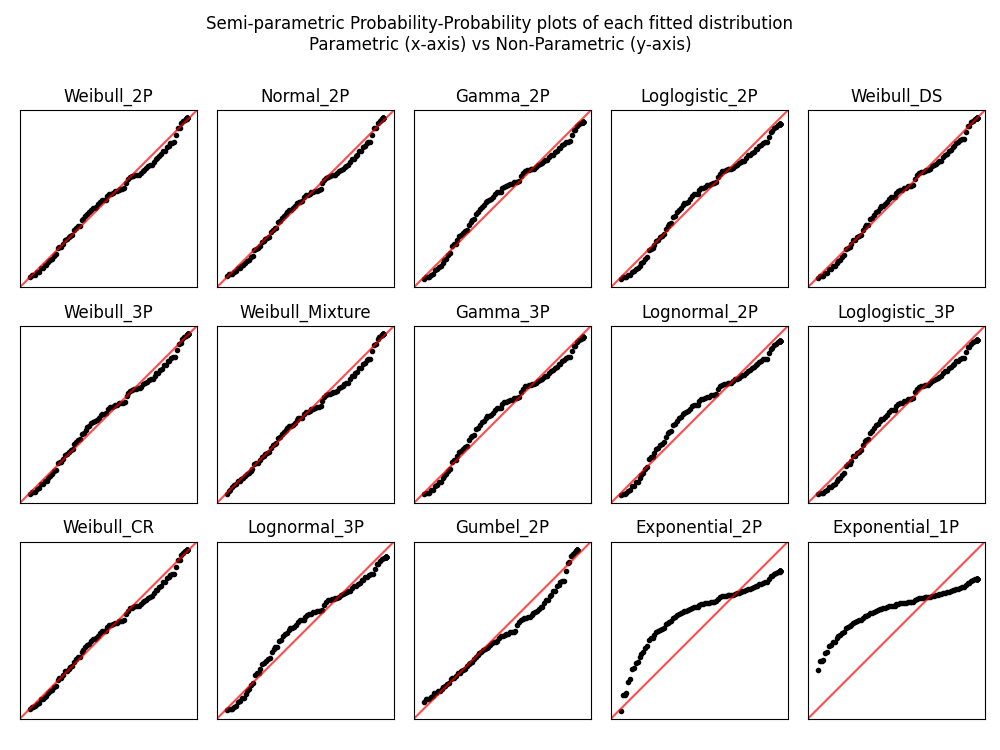# Fitting all available distributions to data¶

To fit all of the distributions available in reliability, is a similar process to fitting a specific distribution. The user needs to specify the failures and any right censored data. The Beta distribution will only be fitted if you specify data that is in the range 0 to 1. The selection of what can be fitted is all done automatically based on the data provided. Manual exclusion of probability distributions is also possible. If you only provide 2 failures the 3P distributions will automatically be excluded from the fitting process.

Inputs:

• failures - an array or list of the failure times.
• right_censored - an array or list of the right censored failure times.
• sort_by - goodness of fit test to sort results by. Must be either ‘BIC’,’AIC’, or ‘AD’. Default is BIC.
• method - ‘LS’ (least squares) or ‘MLE’ (maximum likelihood estimation). Default is ‘MLE’.
• optimizer - ‘L-BFGS-B’, ‘TNC’, or ‘powell’. These are all bound constrained methods. If the bounded method fails, nelder-mead will be used. If nelder-mead fails then the initial guess will be returned with a warning. For more information on optimizers see scipy.
• exclude - list or array of strings specifying which distributions to exclude. Default is None. Options are ‘Weibull_2P’, ‘Weibull_3P’, ‘Normal_2P’, ‘Gamma_2P’, ‘Loglogistic_2P’, ‘Gamma_3P’, ‘Lognormal_2P’, ‘Lognormal_3P’, ‘Loglogistic_3P’, ‘Gumbel_2P’, ‘Exponential_1P’, ‘Exponential_2P’, ‘Beta_2P’
• show_histogram_plot - True/False. Defaults to True. Will show the PDF and CDF of the fitted distributions along with a histogram of the failure data.
• show_PP_plot - True/False. Defaults to True. Provides a comparison of parametric vs non-parametric fit using a semiparametric Probability-Probability (PP) plot for each fitted distribution.
• show_probability_plot - True/False. Defaults to True. Provides a probability plot of each of the fitted distributions.
• print_results - True/False. Defaults to True. Will show the results of the fitted parameters and the goodness of fit tests in a dataframe.

Outputs:

• results - a dataframe of the fitted distributions and their parameters, along with the AICc, BIC, AD, and log-likelihood goodness of fit statistics. This is sorted automatically to provide the best fit first. Use the sort_by=’BIC’ to change the sort between AICc, BIC, AD, and LL. Default sort is BIC. print_results controls whether this is printed. In displaying these results, the pandas dataframe is designed to use the common greek letter parametrisations rather than the scale, shape, location, threshold parametrisations which can become confusing for some distributions.
• best_distribution - a distribution object created based on the parameters of the best fitting distribution. The best distribution is created as a distribution object that can be used like any of the other distribution objects. See the examples below for how this can be used.
• best_distribution_name - the name of the best fitting distribution. E.g. ‘Weibull_3P’
• parameters and goodness of fit results for each fitted distribution. For example, the Weibull_3P distribution values are:
• Weibull_3P_alpha
• Weibull_3P_beta
• Weibull_3P_gamma
• Weibull_3P_BIC
• Weibull_3P_AICc
• Weibull_3P_loglik

Confidence intervals are shown on the plots but they are not reported for each of the fitted parameters as this would be a large number of outputs. If you need the confidence intervals for the fitted parameters you can repeat the fitting using just a specific distribution and the results will include the confidence intervals.

## Example 1¶

In this first example, we will use Fit_Everything on some data and will return only the dataframe of results. Note that we are actively supressing the 3 plots that would normally be shown to provide graphical goodness of fit indications. The table of results has been ranked by BIC to show us that Weibull_2P was the best fitting distribution for this dataset. This is what we expected since the data was generated using Weibull_Distribution(alpha=5,beta=2).

from reliability.Fitters import Fit_Everything
data = [4,4,2,4,7,4,1,2,7,1,4,3,6,6,6,3,2,3,4,3,2,3,2,4,6,5,5,2,4,3] # created using Weibull_Distribution(alpha=5,beta=2), and rounded to nearest int
Fit_Everything(failures=data, show_histogram_plot=False, show_probability_plot=False, show_PP_plot=False)

'''
Results from Fit_Everything:
Analysis method: MLE
Failures / Right censored: 30/0 (0% right censored)

Distribution    Alpha    Beta   Gamma      Mu    Sigma   Lambda  Log-likelihood    AICc     BIC      AD
Weibull_2P  4.21932 2.43761                                          -56.6259 117.696 120.054 1.04805
Gamma_2P 0.816684 4.57133                                          -56.9801 118.405 120.763 1.06592
Normal_2P                          3.73333  1.65193                 -57.6266 119.698 122.056 1.18539
Lognormal_2P                          1.20392 0.503628                 -58.1088 120.662  123.02 1.19881
Weibull_3P  3.61252 2.02388 0.53024                                  -56.4219 119.767 123.047 1.04948
Loglogistic_2P  3.45096 3.48793                                          -58.3223 121.089 123.447  1.0561
Gamma_3P 0.816684 4.57133       0                                  -56.9801 120.883 124.164 1.06592
Lognormal_3P                        0 1.20392 0.503628                 -58.1088 123.141 126.421 1.19881
Loglogistic_3P  3.45096 3.48793       0                                  -58.3223 123.568 126.848  1.0561
Exponential_2P                   0.9999                   0.36584        -60.1668 124.778 127.136 3.11235
Gumbel_2P                          4.58389  1.65599                 -60.5408 125.526 127.884 1.57958
Exponential_1P                                           0.299846        -69.7173 141.578 142.836 5.89119
'''


## Example 2¶

In this second example, we will create some right censored data and use Fit_Everything. All outputs are shown, and the best fitting distribution is accessed and printed.

from reliability.Fitters import Fit_Everything
from reliability.Distributions import Weibull_Distribution
from reliability.Other_functions import make_right_censored_data

raw_data = Weibull_Distribution(alpha=12, beta=3).random_samples(100, seed=2)  # create some data
data = make_right_censored_data(raw_data, threshold=14)  # right censor the data
results = Fit_Everything(failures=data.failures, right_censored=data.right_censored)  # fit all the models
print('The best fitting distribution was', results.best_distribution_name, 'which had parameters', results.best_distribution.parameters)

'''
Results from Fit_Everything:
Analysis method: MLE
Failures / Right censored: 86/14 (14% right censored)

Distribution   Alpha    Beta   Gamma      Mu    Sigma    Lambda  Log-likelihood    AICc     BIC      AD
Weibull_2P 11.2773 3.30301                                           -241.959 488.041 493.128  44.945
Normal_2P                         10.1194  3.37466                  -242.479 489.082 494.169 44.9098
Gamma_2P 1.42314 7.21352                                           -243.235 490.594  495.68 45.2817
Loglogistic_2P 9.86245 4.48433                                           -243.588 491.301 496.387 45.2002
Weibull_3P 10.0786 2.85824 1.15085                                   -241.779 489.807 497.373 44.9927
Gamma_3P 1.42314 7.21352       0                                   -243.235  492.72 500.286 45.2817
Lognormal_2P                         2.26524 0.406436                  -245.785 495.694  500.78 45.6874
Loglogistic_3P 9.86245 4.48433       0                                   -243.588 493.427 500.992 45.2002
Lognormal_3P                       0 2.26524 0.406436                  -245.785  497.82 505.385 45.6874
Gumbel_2P                         11.5926  2.94944                  -248.348 500.819 505.906 45.4624
Exponential_2P                 2.82892                   0.121884        -267.003 538.129 543.216 51.7851
Exponential_1P                                          0.0870024        -295.996 594.034 596.598 56.8662

The best fitting distribution was Weibull_2P which had parameters [11.27730642  3.30300716  0.        ]
'''All plots are ordered based on the goodness of fit order of the results. For the histogram this is reflected in the order of the legend. For the probability plots and PP plots, these are ordered left to right and top to bottom.

The histogram is scaled based on the amount of censored data. If your censored data is all above your failure data then the histogram bars should line up well with the fitted distributions (assuming you have enough data). However, if your censored data is not always greater than the max of your failure data then the heights of the histogram bars will be scaled down and the plot may look incorrect. This is to be expected as the histogram is only a plot of the failure data and the totals will not add to 100% if there is censored data.

Note

The confidence intervals shown on the probability plots are not available for Gamma_2P, Gamma_3P, or Beta_2P. This library is being actively developed the remaining confidence intervals will be added soon.